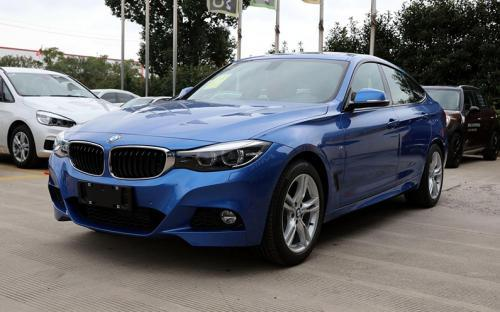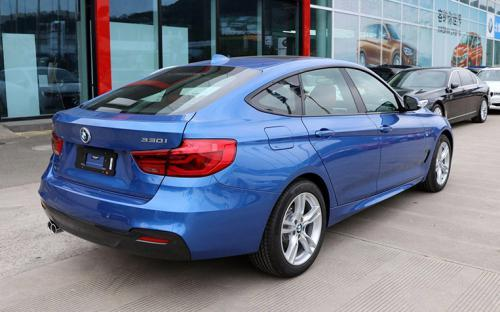宝马(进口) 宝马3系GT2017款最低售价：39.80 万元起

4824(mm)1828(mm)1518(mm)配置亮点：
• 胎压监测装置

• ISOFIX儿童座椅接口

• 车身稳定控制(ESC/ESP/DSC等)

• 电动天窗

• 定速巡航

• 后倒车雷达

• 真皮座椅

• GPS导航系统

• 氙气大灯

• 后视镜加热

• 提交
2017款 xDrive M运动型 (139张)
• 2017款 xDrive M运动型 (139张)
• 2017款 320i M运动型 (131张)
• 宝马(进口) 宝马3系GT 绕车实拍• 宝马(进口) 宝马3系GT 在售车型

排量 车型 厂商指导价 本地最低报价 购车工具
T
320i 时尚型 8挡手自一体
39.80万
320i 豪华设计套装 8挡手自一体
45.40万
320i M运动型 8挡手自一体
45.40万
xDrive M运动型 8挡手自一体
51.90万

宝马(进口) 宝马3系GT 经销商

查看更多 >>

宝马(进口) 宝马3系GT 动力加速

宝马3系GT 0-100公里加速时间分布在 6.2-8.1秒 属于 高性能级

动力级别 加速时间 车型
运动级(1款)
8.1s
320i 时尚型320i 豪华设计套装320i M运动型
高性能级(1款)
6.2s
xDrive M运动型

宝马(进口) 宝马3系GT 视频

宝马(进口) 宝马3系GT 新闻资讯

宝马推出新款3系GT 售价44.5万-69.8万元

进口新车 超过9382次关注

据官方获悉，宝马推出新款3系GT车型，新车共推出6款车型，其中相比老款增加了328ixDrive设计套装型和335ixDrive设计套装型两款四驱版车型，其售价区间为44.5万-69.8万元。

宝马推出新款3系GT 售价44.5万-69.8万元

新闻 超过7847次关注

据官方获悉，宝马将推出新款3系GT车型，共6款车型，新车除最低配320i领先型外，采用纯色细线纹高级木饰的风尚设计套装和炫晶灰色细线纹高级木饰的豪华设计套装，消...

宝马3系GT四驱车型疑似售价 或55.3万起

进口新车 超过5665次关注

日前，据国内媒体报道，宝马将推出3系GT的四驱版车型，新车将推出两款车型，售价或为55.3-69.8万元（注：该售价为网络曝光，具体售价还以官方公布为准）。新车预计...

近期上市跨界车盘点 宝马 3系/GT

导购 超过3770次关注

3系GT的出现是令人震惊的，因为它破坏了宝马的“成长规律”。回想5系GT上市之时，5系Li和5系旅行都已经有了一定的市场后才出现，而3系旅行还未上市，这款3系GT却率...

抢占新的细分市场 宝马3系GT静态评测

试驾评测 超过5306次关注

刚刚在日内瓦车展上高调亮相的全新3系GT，是宝马在全新细分市场的又一次大胆尝试。3系GT衍生于全新一代长轴距3系（F35)平台之上，在功能性和设计风格上结合了旅行车...

诠释另类的优雅 宝马3系GT到店实拍

车系文章 超过5510次关注

日前，宝马公布了3系GT车型的预售价格：45-68万元，上市时间基本确定在6月28日。相信大家之前也从各种渠道了解过这款宝马旗下的“另类”车型，从之前汽车之家关于3...

宝马3系GT有少量现车 本月23日有团购

车系文章 超过2647次关注

近日，编辑从余姚宝恒汽车销售服务有限公司了解到，宝马3系GT店内又少量现车，暂无现金优惠，本月23日店内有团购活动。

宝马3系GT有少量现车销售 看车团招募

车系文章 超过2276次关注

近日，编辑从深圳市驰宝汽车销售服务有限公司，2013款宝马3系GT在店内有少量现车，购车暂无现金优惠。

预售45万起 宝马3系GT将于6月25日上市

车系文章 超过3470次关注

宝马3系GT基于3系长轴距车型平台打造，长宽高分别为4824/1828/1508mm，同时拥有长达2920mm的轴距。此外其后备厢空间也达到520升，当后排座椅折叠放倒后，容积最大可提升至1600升。

猜你喜欢

﻿
• 快速找车
• 选择品牌
• 选择品牌
• A  奥迪
• A  阿斯顿·马丁
• A  阿尔法·罗密欧
• B  宝沃
• B  布加迪
• B  巴博斯
• B  保时捷
• B  宾利
• B  奔驰
• B  宝马
• B  本田
• B  别克
• B  标致
• B  比亚迪
• B  宝骏
• B  北汽制造
• B  北汽新能源
• B  北汽幻速
• B  北汽威旺
• B  北京汽车
• B  奔腾
• B  北汽绅宝
• C  长安
• C  长安商用
• C  长城
• C  昌河
• D  大众
• D  道奇
• D  DS
• D  东南
• D  东风风神
• D  东风风行
• D  东风小康
• D  东风风度
• D  东风
• F  福特
• F  丰田
• F  菲亚特
• F  法拉利
• F  福田
• F  福迪
• F  福汽启腾
• G  观致
• G  广汽传祺
• G  广汽吉奥
• G  GMC
• H  红旗
• H  汉腾汽车
• H  哈弗
• H  哈飞
• H  海格
• H  海马
• H  华颂
• H  黄海
• H  华泰
• H  恒天
• J  吉利汽车
• J  捷豹
• J  Jeep
• J  江淮
• J  江铃
• J  金杯
• J  九龙
• J  金旅
• K  凯翼
• K  凯迪拉克
• K  克莱斯勒
• K  科尼塞克
• K  卡威
• K  开瑞
• L  路虎
• L  林肯
• L  劳斯莱斯
• L  兰博基尼
• L  雷克萨斯
• L  铃木
• L  雷诺
• L  理念
• L  力帆
• L  莲花汽车
• L  猎豹
• L  路特斯
• L  陆风
• M  马自达
• M  MG
• M  MINI
• M  玛莎拉蒂
• M  摩根
• M  迈凯轮
• N  纳智捷
• O  欧宝
• O  讴歌
• O  欧朗
• Q  奇瑞
• Q  起亚
• Q  启辰
• R  日产
• R  荣威
• R  瑞麒
• S  三菱
• S  斯威汽车
• S  萨博
• S  smart
• S  斯柯达
• S  斯巴鲁
• S  思铭
• S  双龙
• S  上汽大通
• S  双环
• T  特斯拉
• T  腾势
• W  沃尔沃
• W  五菱汽车
• W  五十铃
• W  威兹曼
• W  威麟
• X  现代
• X  雪佛兰
• X  雪铁龙
• X  西雅特
• Y  一汽
• Y  英菲尼迪
• Y  英致
• Y  依维柯
• Y  野马汽车
• Y  永源
• Z  众泰
• Z  中华
• Z  中兴
• Z  知豆
• 选择车系
• 选择车系
• 车型对比
• 选择品牌
• 选择品牌
• A  奥迪
• A  阿斯顿·马丁
• A  阿尔法·罗密欧
• B  宝沃
• B  布加迪
• B  巴博斯
• B  保时捷
• B  宾利
• B  奔驰
• B  宝马
• B  本田
• B  别克
• B  标致
• B  比亚迪
• B  宝骏
• B  北汽制造
• B  北汽新能源
• B  北汽幻速
• B  北汽威旺
• B  北京汽车
• B  奔腾
• B  北汽绅宝
• C  长安
• C  长安商用
• C  长城
• C  昌河
• D  大众
• D  道奇
• D  DS
• D  东南
• D  东风风神
• D  东风风行
• D  东风小康
• D  东风风度
• D  东风
• F  福特
• F  丰田
• F  菲亚特
• F  法拉利
• F  福田
• F  福迪
• F  福汽启腾
• G  观致
• G  广汽传祺
• G  广汽吉奥
• G  GMC
• H  红旗
• H  汉腾汽车
• H  哈弗
• H  哈飞
• H  海格
• H  海马
• H  华颂
• H  黄海
• H  华泰
• H  恒天
• J  吉利汽车
• J  捷豹
• J  Jeep
• J  江淮
• J  江铃
• J  金杯
• J  九龙
• J  金旅
• K  凯翼
• K  凯迪拉克
• K  克莱斯勒
• K  科尼塞克
• K  卡威
• K  开瑞
• L  路虎
• L  林肯
• L  劳斯莱斯
• L  兰博基尼
• L  雷克萨斯
• L  铃木
• L  雷诺
• L  理念
• L  力帆
• L  莲花汽车
• L  猎豹
• L  路特斯
• L  陆风
• M  马自达
• M  MG
• M  MINI
• M  玛莎拉蒂
• M  摩根
• M  迈凯轮
• N  纳智捷
• O  欧宝
• O  讴歌
• O  欧朗
• Q  奇瑞
• Q  起亚
• Q  启辰
• R  日产
• R  荣威
• R  瑞麒
• S  三菱
• S  斯威汽车
• S  萨博
• S  smart
• S  斯柯达
• S  斯巴鲁
• S  思铭
• S  双龙
• S  上汽大通
• S  双环
• T  特斯拉
• T  腾势
• W  沃尔沃
• W  五菱汽车
• W  五十铃
• W  威兹曼
• W  威麟
• X  现代
• X  雪佛兰
• X  雪铁龙
• X  西雅特
• Y  一汽
• Y  英菲尼迪
• Y  英致
• Y  依维柯
• Y  野马汽车
• Y  永源
• Z  众泰
• Z  中华
• Z  中兴
• Z  知豆
• 选择车系
• 选择车系
• 选择车型
• 选择车型
• 意见反馈